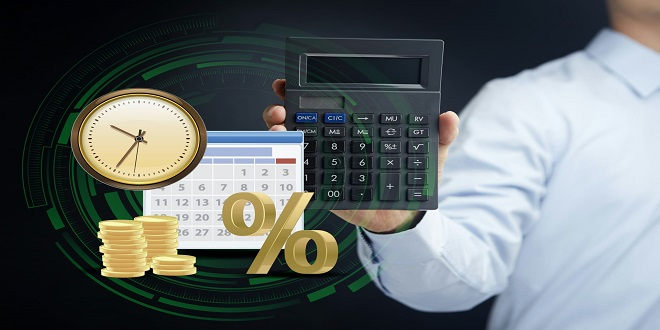# A Conclusive Guide on How to Use a FD Return Calculator

In the last few years, India has witnessed a growth in the amount that people are investing as fixed deposit. In the FY 2019, this amount stood at 44.18 trillion INR. It increased by around 2 trillion INR in the FY 2020 with the total amount of FDs in India hitting 46 trillion INR!  The popularity of FD can be traced to how it is not tied with the market, making it a very stable and safe option of investing money. In addition to this, the terms of an FD are flexible, and one can avail tax deductions and loans on the FD.

In this article, we seek to help you understand all you need to know about an FD return calculator. These calculators are fully automated tools available online, that can be used to calculate the amount you will receive at the end of the tenure of your fixed deposit.

Before we delve into FD returns and its calculations, let us answer the question, “What is fixed deposit?” A fixed deposit is a basic investment opportunity offered by banks and  NBFCS and banks to enable customers to save a significant portion of their funds. These funds will then grow according to the interest rate that has been previously agreed upon. Upon maturity of the FD, the customer becomes entitled to FD returns – the original sum invested along with the interest accrued on it.

What affects FD returns?

Before we get into the usage of an FD return calculator, it is important to understand the factors that affect these returns. Here are a few factors that significantly affect FD returns.

• The Principal Amount – It goes without saying that the amount invested into the FD will determine the returns, the greater the principal amount, the greater the FD return will be.
• The Rate of Interest – The growth of your money in an FD increases with a higher rate of interest on FD.
• The Deposit Type – FDs can be cumulative (compounded 4 times a year and received upon maturity) and those that are non-cumulative (interest is paid periodically according to your preference). Cumulative deposits reap higher returns as the interest is further invested as it accrues, until maturity.
• Your Age – If you are a senior citizen, you are eligible for higher rate of interest and therefore higher FD returns, than non-senior citizens.

Formulae Used to Calculate FD Returns

FD returns calculators use two formulae depending on whether the interest on the FD is simple or compound.

• FD returns for simple interest: M = P + (P*R*T / 100)

In this formula, M refers to the FD return being calculated, P is the principal amount, R the rate of interest per annum, and T is the tenure of the fixed deposit. Say for instance, Rs. 10,000 is deposited for a period of 5 years at an interest rate of 5% per annum. Then the FD return will be,

M = 10,000 + (10,000 * 5 * 5/100)

M = 10,000 + 2500

M= Rs. 12,500

• FD returns for compound interest: M = P + P {(1 + i / 100) t – 1}

Here also, M and P refer to the FD return and principal amount, respectively. ‘t’ refers to the duration of the fixed deposit, and ‘i’ refers to the rate of interest per period. Let us look at an example to understand this formula better. Consider a deposit amount of Rs. 10,00,000 (Rupees ten lakhs) which is at a rate of 10% per annum and exists for 5 years. In such a case, the return calculated by the FD return calculator will be as under:

M = 10,00,000 + {(1 + 10/100) 5 – 1}

M = Rs. 10,61,051

How to use an online FD return calculator?

Using an online FD maturity calculator is an easy task and takes less than a minute. All you have to do is visit the website of your insurance company. Then, search for the FD calculator. Enter into the FD calculator information like the Principal Amount, the Rate of Interest and the Tenure of the FD you are looking at. In a matter of seconds, the FD returns you will receive upon maturity will be displayed on the screen!

Why use an online FD return calculator?

It goes without saying that using an online FD return calculator simplifies the process of calculation by leaps and bounds. You will receive your answer just as you finish entering the details on the calculator. Additionally, as the entire process is automated there is no scope for error and you can rest assured that the amounts displayed by the maturity calculator will always be accurate. Furthermore, knowing in advance what the returns on the investment will be helps make sound choices like the amount to be deposited, the rate of interest to be agreed upon, the tenure that will give you the best results, etc. Over and above this, you can use FD return calculators to compare the returns you will receive from different companies, and you can choose the best one to invest your money in.